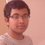# Interesting Integral

I found this result while studying indefinite integral calculus last year.

$\displaystyle I_{n}\,=\, \int e^{t} \cdot \left[f(t)+(-1)^{n-1}\cdot f^{(n)}(t)\right]dt\,=\,e^{t}\cdot\left[f(t)-f^{\prime}(t)+f^{\prime \prime}(t)-...+(-1)^{n-1}f^{(n-1)}(t)\right]+c$, where $f^{(k)}(x)$ denotes the $k$th derivate of $f(x)$ and $n \,\in\, \mathbb{Z^{+}}$.

This is basically the generalization of the integral $\displaystyle \int e^{t} \cdot \left[f(t)+f^{\prime}(t)\right] dt\,=\,e^{t}\cdot f(t)\,+\,c$.

$\huge \text{Proof :-}$

It can be seen that $\displaystyle I_{1}\,=\, \int e^{t} \cdot \left[f(t)+f^{\prime}(t)\right] dt$. Using integration by parts,

$\displaystyle I_{1}\,=\, \int e^{t} \cdot \left[f(t)+f^{\prime}(t)\right] dt\,=\,\int \underbrace{e^{t}}_{\text{II}}\cdot \underbrace{f(t)}_{\text{I}} \,dt + \int e^{t} \cdot f^{\prime}(t) \,dt \,=\,e^{t}\cdot f(t) \,-\, \int e^{t} \cdot f^{\prime}(t)\,dt+ \int e^{t} \cdot f^{\prime}(t)\,dt\,=\,e^{t}\cdot f(t) + c $/extract_itex]. So, $I_{1}$ is true. Assume, $I_{r}$ is true i.e $\displaystyle I_{r}\,=\, \int e^{t} \cdot \left[f(t)+(-1)^{r-1}\cdot f^{(r)}(t)\right]dt\,=\,e^{t}\cdot\left[f(t)-f^{\prime}(t)+f^{\prime \prime}(t)-...+(-1)^{r-1}f^{(r-1)}(t)\right]+c$. Consider, $I_{r+1}\,=\,\int e^{t} \cdot \left[f(t)\,+\,(-1)^{r} \cdot f^{(r+1)}(t)\right]\,dt\,=\,\int e^{t} \cdot \left[f(t)\,+\,\mathbin{\color{#3D99F6}(-1)^{r-1} \cdot f^{(r)}(t)}\,-\,\mathbin{\color{#3D99F6}(-1)^{r-1} \cdot f^{(r)}(t)} \,+\,(-1)^{r} \cdot f^{(r+1)}(t)\right]\,dt$ $\implies\int e^{t} \cdot \left[f(t)\,+\,\mathbin{\color{#3D99F6}(-1)^{r-1} \cdot f^{(r)}(t)}\,+\,\mathbin{\color{#3D99F6}(-1)^{r} \cdot f^{(r)}(t)} \,+\,(-1)^{r} \cdot f^{(r+1)}(t)\right]\,dt\,=\, \int e^{t} \cdot \left[f(t)\,+\,\mathbin{\color{#3D99F6}(-1)^{r-1} \cdot f^{(r)}(t)}\right]dt \,+\, \int e^{t} \cdot \left[\mathbin{\color{#3D99F6}(-1)^{r} \cdot f^{(r)}(t)} \,+\,(-1)^{r} \cdot f^{(r+1)}(t)\right]\,dt$ $\implies I_{r}\,+\, (-1)^{r} \cdot \int \left[\underbrace{e^{t}}_{\text{II}}\cdot \underbrace{\mathbin{\color{#3D99F6} f^{(r)}(t)}}_{\text{I}} \,+\,e^{t}\cdot f^{(r+1)}(t)\right]\,dt\,=\,I_{r}+ (-1)^{r} \cdot \left[e^{t} \cdot f^{(r)}(t)\,-\,\int e^{t} \cdot f^{(r+1)}(t)\,dt\,+\,\int e^{t} \cdot f^{(r+1)}(t)\,dt\right]\,=\,I_{r}+(-1)^{r} \cdot e^{t}\cdot f^{(r)}(t)$. From our assumption, it follows that, $I_{r+1}\,=\,e^{t}\cdot\left[f(t)-f^{\prime}(t)+f^{\prime \prime}(t)-...+(-1)^{r}f^{(r-1)}(t)+(-1)^{r}\cdot f^{(r)}(t)\right]+c$. So, $I_{r+1}$ is true. Hence, $I_{r}$ is true $\implies$ $I_{r+1}$ is true. So, by induction, $I_{n}$ is true $\forall \,n \,\in\,\,\mathbb{Z^{+}}$. Also, integrals of the form $\displaystyle \int \left[f(\log x)+(-1)^{n-1}\cdot f^{(n)}(\log x)\right]\,dx$ can be reduced to the aforementioned integral by the substitution $t=\log (x)$.Note by Aditya Sky 4 years, 2 months ago This discussion board is a place to discuss our Daily Challenges and the math and science related to those challenges. Explanations are more than just a solution — they should explain the steps and thinking strategies that you used to obtain the solution. Comments should further the discussion of math and science. When posting on Brilliant: • Use the emojis to react to an explanation, whether you're congratulating a job well done , or just really confused . • Ask specific questions about the challenge or the steps in somebody's explanation. Well-posed questions can add a lot to the discussion, but posting "I don't understand!" doesn't help anyone. • Try to contribute something new to the discussion, whether it is an extension, generalization or other idea related to the challenge. • Stay on topic — we're all here to learn more about math and science, not to hear about your favorite get-rich-quick scheme or current world events. MarkdownAppears as *italics* or _italics_ italics **bold** or __bold__ bold - bulleted- list • bulleted • list 1. numbered2. list 1. numbered 2. list Note: you must add a full line of space before and after lists for them to show up correctly paragraph 1paragraph 2 paragraph 1 paragraph 2 [example link](https://brilliant.org)example link > This is a quote This is a quote  # I indented these lines # 4 spaces, and now they show # up as a code block. print "hello world" # I indented these lines # 4 spaces, and now they show # up as a code block. print "hello world" MathAppears as Remember to wrap math in $$ ... $$ or \[ ... $ to ensure proper formatting.
2 \times 3 $2 \times 3$
2^{34} $2^{34}$
a_{i-1} $a_{i-1}$
\frac{2}{3} $\frac{2}{3}$
\sqrt{2} $\sqrt{2}$
\sum_{i=1}^3 $\sum_{i=1}^3$
\sin \theta $\sin \theta$
\boxed{123} $\boxed{123}$

Sort by:

Awesome proof - dont have time to check its validity but quick glance looks correct!

ground braking

- 3 years ago